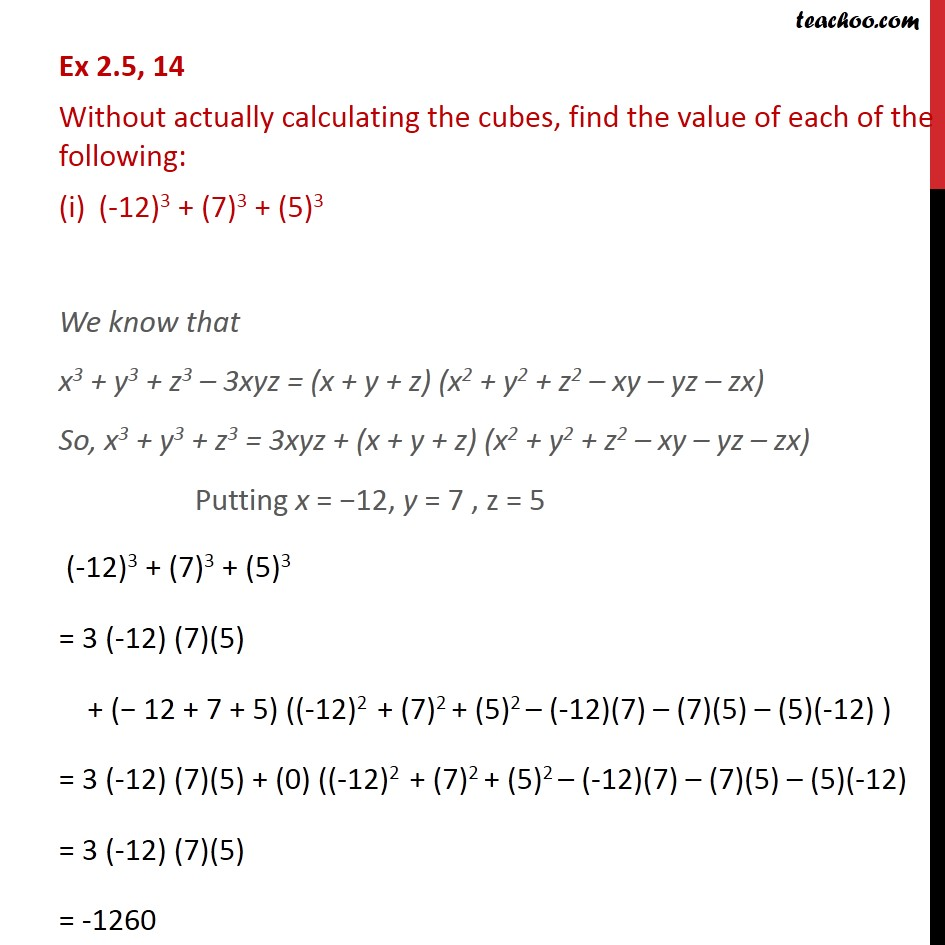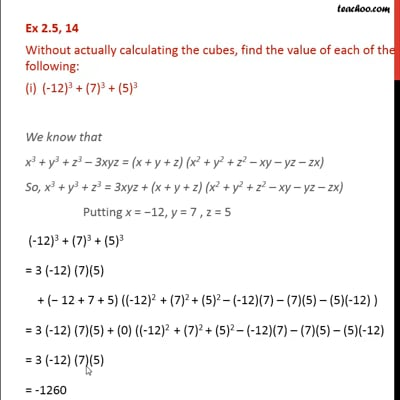Ex 2.5

Chapter 2 Class 9 Polynomials
Serial order wiseThis video is only available for Teachoo black users

Solve all your doubts with Teachoo Black (new monthly pack available now!)

### Transcript

Ex 2.5, 14 Without actually calculating the cubes, find the value of each of the following: (-12)3 + (7)3 + (5)3 We know that x3 + y3 + z3 3xyz = (x + y + z) (x2 + y2 + z2 xy yz zx) So, x3 + y3 + z3 = 3xyz + (x + y + z) (x2 + y2 + z2 xy yz zx) Putting x = 12, y = 7 , z = 5 (-12)3 + (7)3 + (5)3 = 3 (-12) (7)(5) + ( 12 + 7 + 5) ((-12)2 + (7)2 + (5)2 (-12)(7) (7)(5) (5)(-12) ) = 3 (-12) (7)(5) + (0) ((-12)2 + (7)2 + (5)2 (-12)(7) (7)(5) (5)(-12) = 3 (-12) (7)(5) = -1260